Hi everyone in this article I’m explaining about angularjs expressions.

Introduction:

AngularJS allows the following two different ways to bind data to HTML.

1.       By using ng-bind directive.

2.      By using expressions.

We have already gone through directives in previous articles in which we discussed the ng-bind directive. Dealing with expressions is the purpose of this article.

AngularJS Expressions:

Expressions are used to bind the data to HTML (a view) and also decides the position of that data, how and where it should be displayed in the view. AngularJS expressions are defined inside the the double curly braces, for example {{expression here}}.

Like JavaScript expressions, AngularJS expressions can also have various valid expressions. We can use operators between numbers and strings, literals, objects and array’s inside the expression {{ }}. For example:

{{ 2 + 2 }} (numbers)

{{Name + " " + email}} (string)

{{ Country.Name }} (object)

{{ fact }} (array)

AngularJs Expression vs JavaScript Expression:

1.   In JavaScript, if we try to evaluate the properties that are not previously defined then JavaScript generates a RefrenceError or TypeError whereas for AngularJS we will get undefined or null.

2.  In JavaScript, a control flow statement can be used whereas we cannot use one in AngularJS.

3.  There is not the tradition of using a comma or void in an AngularJS expression.

4.  To set the format of data, we can use a filter with an AngularJS expression, like {{expression| filter}} {{number | currency}} will format the number as a currency like \$number. For example {{100|currency}} will reduce \$100.00. We have more filters in AngularJS like date, filter, json, and limit to, lowercase, number, and order by and uppercase.

Let's go through a simple example. We will first see how to bind data using the ng-bind directive then we will move to the main agenda of this article, AngularJS expressions.

<div ng-app="" ng-init="firstName='Kamlakar';lastName='Singh'">
<h1>Without using expression</h1>
Fullname : <span ng-bind="firstName +' ' + lastName "></span> //ng-bind using
</div>

Output: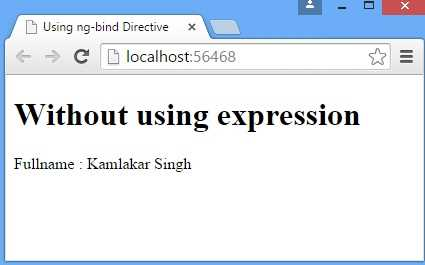For the same piece of the preceding code, we can bind the data by replacing ng-bind by {{expression}}. Here is how it is.

<div ng-app="" ng-init="firstName='Kamlakar';lastName='Singh'">
<h1>using expression</h1>
Fullname : {{firstName+''+lastName}} //expression
</div>

Output: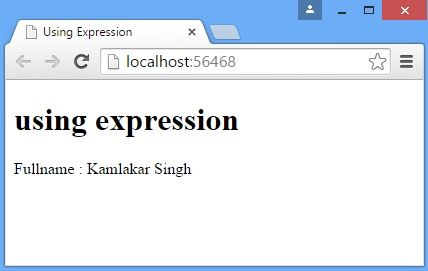The preceding example showed the use of a string inside an expression, just like:

{{Name + " " + email}} (string)

Using Filter in Expression:

<div ng-app="" ng-init="firstName='Kamlakar';lastName='Singh'">
<h1>using expression|uppercase</h1>
Fullname : {{firstName+''+lastName|uppercase}}
<!--//using filter uppercase-->
</div>
Output: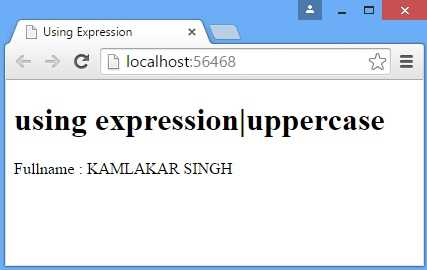Similarly we can apply various types of filters depending on our needs.

Binding and Displaying AngularJS Number:

As explained about JavaScript expression and AngularJS expressions earlier, we can use numbers inside AngularJS expressions that are just like JavaScript numbers in which we can filter using a pipe '|' character.

<h1>Number|currency</h1>
<div ng-app="" ng-init="items=5;price=45.50">
<p>Total Price: {{ items * price|currency }}</p>
</div>

Output: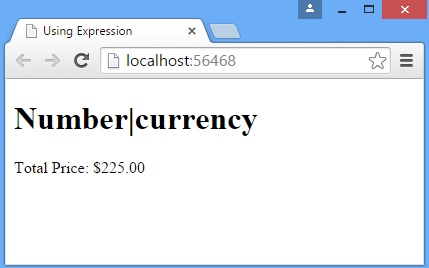Binding and Displaying AngularJs Object:

We can also use AngularJS objects just like we did with JavaScript objects.These objects are used inside an expression.

<div ng-app="" ng-init="employee={name:'Kamlakar',Role:'Software Developer'}">
<p>{{ employee.name }} Singh is a {{employee.Role}}</p>
</div>

Output: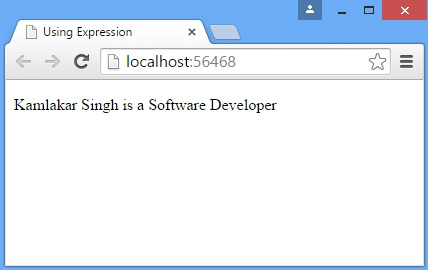Working with Arrays in Angularjs:
AngularJS arrays behave just like we studied in JavaScript. Let's understand with a simple demo example.

<div ng-app="" ng-init="prime=[2,3,5,7,11,13]">
<p>Fourth primevalue is: {{ prime }}</p>
</div>

Output: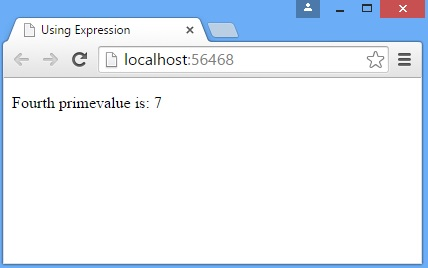Modified On Sep-07-2019 12:15:27 AM